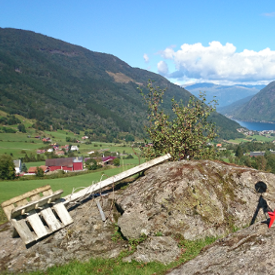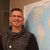# Transform MANUALLY WGS84 to NAD83 CSRS

611
4
12-22-2020 07:24 AM
Labels (1)by
New Contributor

Hi,
I wanted to use the coordinate frame rotation method to transform MANUALLY WGS84 to NAD83 CSRS. Some of the parameters to do so are given in meter (dx, dy and dz). I tried many times to do this transformation using the formulas for this method. But no result concords with the values given by ArcGIS when I do this transformation using this software.
could you please tell me if I have to transform some parameters in other units before applying the formulas.

lat/long of my point in WGS84: LAT=47.562060394000000 LONG=-52.740740910000000

formulas:
M=(1+dS*10^-6)
XT=M*(1*Xs-Rz*Ys+Ry*Zs)+dx
YT=M*(Rz*Xs+1*Ys-Rx*Zs)+dy
ZT=M*(-Ry*Xs+Rx*Ys+1*Zs)+dz

parameters: dx=0.991000000, dy=-1.907200000, dz=-0.512900000, rx (second)=0.02578991, ry (second)=0.00965010, rz (second)=0.01165994, ds=0.000000000

Expected (ARCGIS) - From WGS84 to NAD83_CSRS:
LAT=47.562050544
LONG=-52.740746617

4 RepliesOccasional Contributor

Hi. One thing which sometimes cause issues are the signs/orientations, i.e a positive angle can be clockwise or counterclockwise. The R's are rotation matrixes and programmatically you would convert the r's to radians prior to compute the needed cos(r) and sin(r)byEsri Contributor

Hi @akisai,

Transformation parameters you are using match NAD_1983_CSRS_To_WGS_1984_2 (WKID::1946) transformation, but they have opposite +/- signs. From your formulas, I see your rotation matrix matches the one for Position Vector and not the Coordinate Frame method. In your case, you are trying to apply the transformation in reverse (from WGS84 to NAD83 CSRS) by using transformation parameters and rotation matrix with opposite signs as they are originally defined. I would need to verify the math first, but I suspect your approach might not match the exact reverse formulas used in ArcGIS.

Please see EPSG Guidance Note 7.2 for detailed (exact reverse) formulas and the table of Geographic Transformations in ArcGIS Pro 2.7.

I hope this helps...

Tags (2)by
New Contributor

Hi